Courses

# Oxidation Number Class 11 Notes | EduRev

## Class 11 : Oxidation Number Class 11 Notes | EduRev

The document Oxidation Number Class 11 Notes | EduRev is a part of the Class 11 Course Chemistry for JEE.
All you need of Class 11 at this link: Class 11

Oxidation Number

The oxidation number is defined as the charge in which an atom appears to have when all other atoms are removed from it as ions. It may have + or – sign.

[An element may have different values of oxidation number depending upon the nature of compound in which it is present.]

Oxidation number of an element may be a whole number (positive or negative) or fractional or zero.

Important Points for Determining Oxidation Number

1. The algebraic sum of the oxidation numbers of all the atoms in an uncharged (neutral) compound is zero. In an ion, the algebraic sum is equal to the charge on the ion.
2.  All elements in the elementary state have oxidation number zero, e.g., He, Cl2, S8, Petc.
3.  As fluorine is the most electronegative element, it always has an oxidation number of – 1 in all of its compounds.
4.  In compounds containing oxygen, the oxidation number of oxygen is – 2 except in peroxides (-1) such as Na2O2, in OF2 and in O2F2 (+2 and + 1 respectively).
5. In all compounds, except ionic metallic hydrides, the oxidation number of hydrogen is +1. In metal hydrides like NaH, MgH2, CaH2, LiH, etc the oxidation number of hydrogen is -1.
6. Oxidation number for alkali metals is +1 and for alkaline earth metals is +2.
7.  Oxidation number of metal in amalgams is zero.
8.  In case of coordinate bond, it gives +2 value of oxidation number to less electronegative atom and -2 value to more electronegative atom.
9. If coordinate bond is directed from more electronegative to less electronegative atom then its contribution be zero for both the atoms.
10. For p-block elements [Except F and O], the highest oxidation number is equal to their group number and lowest oxidation number is equal to the group number minus eight.
11. In transition elements, the lowest oxidation number is equal to the number of ns electrons and highest oxidation number is equal to number of ‘ns’ and (n – l)d unpaired electrons.

Determination of Oxidation Number of Underlined Element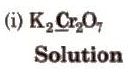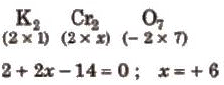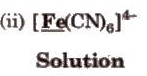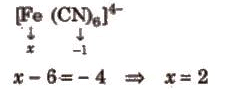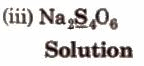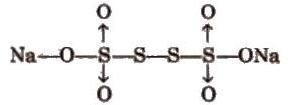Oxidation number of Na = + 1

Oxidation number of 0 = – 2

∴ 2 (1) + 4x + 6 x – 2 = 0

a = 5 / 2, this is average oxidation number, because the compound has two types of sulphur atom.

OX of sulphur bonded with coordinate bond = 5

OX of sulphur which have S-S bond = 0

Average oxidation number = 5 + 5 + 0 + 0 / 4 = 5 / 2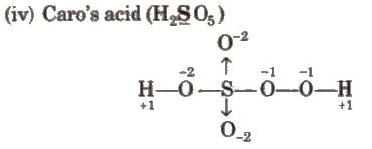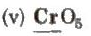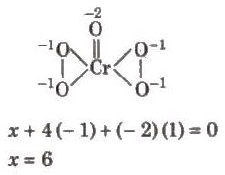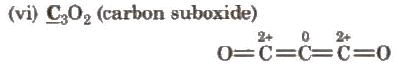(vii) NH4 NO3

There are two types of nitrogen atoms. Therefore, evaluation should be made separately as

Oxidation number of N in NH+4 is,

x + 4 (+ 1)= + 1

x = – 3

Oxidation number of N in NO3 is,

y + 3 x (- 2) = – 1

y = 5

Stock Notations

The oxidation states of elements exhibiting variable oxidation states are specified by Roman numerals such as I, II, III, IV, etc., within parenthesis after the symbol or name of the element.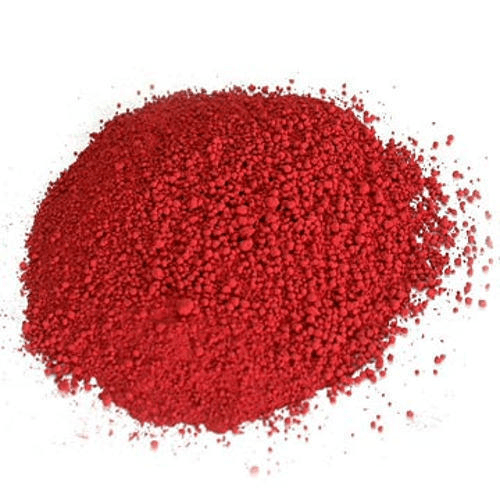Fig: Cuprous oxide powder

This system was introduced for the first time by a German chemist, Alfred Stock and is known as Stock notation. This may be illustrated as:

 Formula of the compounds Chemical name Stock notation Cu20 Cuprous oxide Copper (i) oxide; Cu2(i)0 Fe2O3 Ferric oxide iron (IlI) oxide; Fe2(lII)03 HgCI2 Mercuric chloride Mercury (M) chloride; Hg(lI) Cl2

Offer running on EduRev: Apply code STAYHOME200 to get INR 200 off on our premium plan EduRev Infinity!

## Chemistry for JEE

223 videos|452 docs|334 tests

,

,

,

,

,

,

,

,

,

,

,

,

,

,

,

,

,

,

,

,

,

;